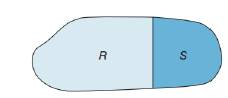Chapter 8.1, Problem 43E### Elementary Geometry for College St...

6th Edition
Daniel C. Alexander + 1 other
ISBN: 9781285195698

#### Solutions

Chapter
Section### Elementary Geometry for College St...

6th Edition
Daniel C. Alexander + 1 other
ISBN: 9781285195698
Textbook Problem
1 views

# Given region R ∪ S , explain why A R ∪ S > A R .To determine

To explain:

The region ARS>AR if the region RS.

Explanation

Given,

From the figure, region R and S are the mutually exclusive and exhaustive.

(i.e.) RS

Where RS=R+S

Area of the region R and S is AR and AS.

Thus, the area of the figure is ARS

(i.e.) ARS=AR+S

### Still sussing out bartleby?

Check out a sample textbook solution.

See a sample solution

#### The Solution to Your Study Problems

Bartleby provides explanations to thousands of textbook problems written by our experts, many with advanced degrees!

Get Started

#### Graphing Inequalities Graph the inequality. 98. y x2 3

Precalculus: Mathematics for Calculus (Standalone Book)

#### Evaluate the limit, if it exists. limx164x16xx2

Single Variable Calculus: Early Transcendentals

#### In Exercises 1-22, evaluate the given expression. P(5,3)

Finite Mathematics for the Managerial, Life, and Social Sciences